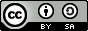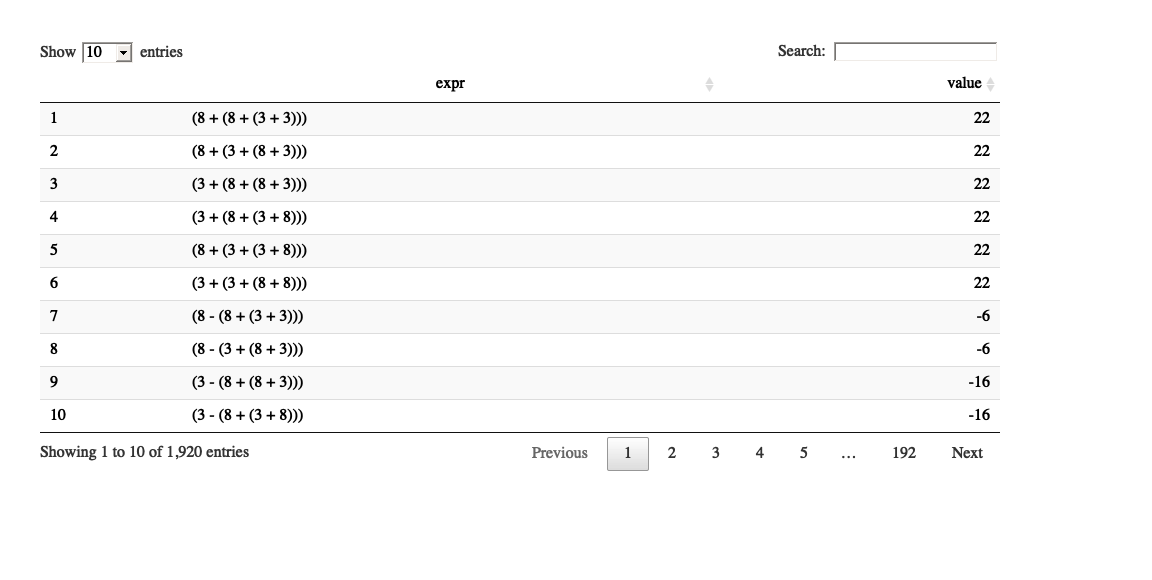[This article was first published on Theory meets practice..., and kindly contributed to R-bloggers]. (You can report issue about the content on this page here)
Want to share your content on R-bloggers? click here if you have a blog, or here if you don't.

## Abstract:

We use the purrr package to solve a popular math puzzle via a combinatorial functional programming approach. A small shiny app is provided to allow the user to solve their own variations of the puzzle.This work is licensed under a Creative Commons Attribution-ShareAlike 4.0 International License. The markdown+Rknitr source code of this blog is available under a GNU General Public License (GPL v3) license from github.

## Introduction

No. 4 of the Top 5 hard math puzzles at briddles.com goes like this:

How can I get the answer 24 by only using the numbers 8,8,3,3. You can use the main signs add, subtract multiply and divide.

Note: a solution has to use each of the specified 4 numbers exactly ONCE, but they can be used in any order. In other words the standard scheme is to solve expressions of the kind:

a op1 b op2 c op3 d

where a, b, c and d denote a permutation of the numbers 8, 8, 3, 3 and each of op1, op2 and op3 denotes the use of one binary operator selected from +, -, * or /. An example is the expression 8 + 3 + 8 * 3. Parentheses are used to control the order in which the operators are applied, i.e. (8 + 3 + 8) * 3 yields a different result than 8 + 3 + (8 * 3).

After a few unsuccessful attempts to solve the above puzzle with pen and paper it felt more efficient and computationally challenging to solve this puzzle via a combinatorial approach: Simply try out all permutations of the 4 numbers, the 3 binary operators and all possible sets of parentheses to combine the operators. One can show that there are at most

\begin{align*} && \text{# permutations of the k=4 base numbers} \\ \times && \text{# ways to select with replacement (k-1) binary operators from the set \{+,-,*,/\} }\\ \times && \text{# ways to parenthesize the (k-1) binary operators} \\ &=&k! \times 4^{(k-1)} \times \frac{1}{k} \binom{2k-2)}{k-1} \end{align*}

different combinations to choose from.1 As an example: for $$k=4$$ the maximal number of unique combinations is 21504.

#### Strategy

We will use a functional approach to solve the above combinatorial problem. Why?

• because it seems like a good use-case for functional programming,
• because it is important to extend your programming horizon every once in a while, and
• because the purrr functional programming toolkit for R allows you to experiment with this without having to leave the R universe.2

For those not familar with purrr can find a wonderful didactic introduction in the useR! 2017 tutorial by Charlotte Wickham. Furthermore, learning purrr was the 7th most frequent mentioned package in the #rstats users’ 2019 R goals. In other words: Attention #rstats new years resolution makers: reading this post is as obligatory as going to the gym on 01 Jan!

## Solving the Math Puzzle

We will divide-and-conquer the solution along the lines of the number of combinations formula: Firstly, we will store all permutations of the $$(k-1)$$ base numbers in a list perm. Secondly, we will store all possible combinations of the $$(k-1)$$ operators in a list operators and, thirdly, we generate all possible ways of putting parentheses around the operators into a list brackets. Subsequently, we form the Cartesian product of these three lists and build the corresponding expression for each triple of permutation, operators and parentheses. Finally, each generated expression is evaluated. The entire result is a data frame containing all possible expressions and their associated value obtained when evaluating the expression.

### Permutations of the base numbers

We let the variable base_numbers contain the specification of the numbers to permute for the expression. The code should be written general enough so it is possible to use a different base, e.g., $$k=3$$ or $$k=5$$.

base_numbers <- c(8,8,3,3)
k <- length(base_numbers)

number_perm <- combinat::permn(base_numbers) %>%
map(setNames, nm=letters[seq_len(k)])

##Slim in case permutations of the base numbers contain duplicates.
perm <- number_perm[!duplicated(map(number_perm, paste0, collapse=""))]

For $$k=4$$ this makes a total 21504 combinations. However, since the numbers 8 and 3 both appear more than once in the base numbers, we can slim the number of permutations from 24 to 6. Hence, there are altogether only 5376 combinations to investigate.

### Combinations of the operators

The next step is to make all combinations of the $$k-1$$ binary operators needed to combine the $$k$$ numbers. We use the string format to represent the operators3 and thus just need the $$k-1$$'th Cartesian product of the set $$\{+, -, *, /\}$$ represented as strings.

opList <- list("+", "-", "*", "/")
##Repeat the opList k-1 times
opsList <- map( seq_len(k-1), ~ opList)
##Form the Cartesian product
operators <- cross(opsList) %>%
map( setNames, nm=paste0("op",seq_len(k-1)))

### Arrangements of the parentheses

As all the involved operators are binarym it becomes clear that finding all possible ways to parenthesize the expression corresponds to finding all binary trees with $$k-1$$ leaves. Beautiful recursive code inspiration for how to solve this can be found on leetcode.com. Some adaptation to R and our problem at hand was necessary - the idea is to use recursion in $$k$$ and use a hash-map to cache results of previous computations.

##Initialize hashmap to save the results of all binary trees up to n=1 leaves
trees <- list()
trees[["0"]] <- NULL
trees[["1"]] <- list(list(val="node", left=NULL, right=NULL))

The rather elegant recursive solution to generate all binary trees with $$n$$ leaves works combining all possible ways to combine subbranches containing $$x$$ and $$n-x$$ leaves, respectively:

allBinTrees <- function(n) {
##Character version of n, which is used as hash key
n_char <- as.character(n)

##Only compute something if n is not already in the tree list.
if (is.null(pluck(trees, n_char))) {
trees[[n_char]] <<- list()
for (i in 1:(n-1)) {
j = n - i
for (left_tree in allBinTrees(i)) {
for (right_tree in allBinTrees(j)) {
trees[[n_char]][[length(trees[[n_char]]) + 1]] <<- list(val=NULL, left=left_tree, right=right_tree)
}
}
}
} #end if not already in tree list
##Return result from our hashmap
return(pluck(trees, n_char))
}

We can test the function for $$n=2$$, which yields exactly one tree:

##Manual construction
trees2 <- list(list(val=NULL, left=trees[["1"]][], right=trees[["1"]][]))
all.equal(allBinTrees(n=2), trees2)
##  TRUE

The result is:

tree2String(allBinTrees(n=2)[]) %>% replaceNodes() %>% addOpNumbers
##  "(a op1 b)"

In the above code segments the function tree2String is a small helper function to convert the nested list structure to a string - in this case: (node op node). Furthermore, the function replaceNodes renames the terms node into the variables (a op b). The op-strings are converted into numbered op-strings using addOpNumbers, i.e. the result becomes (a op1 b). Details about the helper functions can be found in the code on github.

With all preparations in place we can now generate all 5 possible ways to parenthesize the 3 binary operators using the following code:

##Make all possible brackets
bracketing <- map_chr( allBinTrees(n=k),
~ tree2String(.x) %>% addOpNumbers %>% replaceNodes)
##  "(a op1 (b op2 (c op3 d)))" "(a op1 ((b op2 c) op3 d))" "((a op1 b) op2 (c op3 d))" "((a op1 (b op2 c)) op3 d)" "(((a op1 b) op2 c) op3 d)"

### Putting it all together

We can now generate all combinations of numbers, operators and bracketing by the Cartesian of the three lists:

combos <- cross3( perm, map( operators, unlist), bracketing) %>%
map(setNames, c("numbers", "operators", "bracket"))

We can now finally evaluate each of the 1920 combinations. Note: Because this might take a while it's a good idea to add a progress bar for this purrr computation.

##Set up a progress bar for use with the map function
pb <- progress_estimated(length(combos))

##Compute
res <- map(combos, .f=function(l) {
pb$tick()$print()
l[["expr"]] <- l[["bracket"]] %>% replace(l[["numbers"]]) %>% replace(l[["operators"]])
l[["value"]] <- eval(parse(text=l[["expr"]]))
return(l)
})

Again, replace(v) is a small helper function to replace the strings in names(v) with v's content. The actual evaluation of each possible solution string is done by parsing the string with parse and then evaluate the resulting expression. We extract the relevant results into a data.frame

df <- map_df(res, ~ data.frame(expr=.x$expr, value=.x$value))We can now easily extract the solution:

##First element to give the value 24
detect(res, ~ isTRUE(all.equal(.x$value, 24))) ##$numbers
## a b c d
## 8 3 8 3
##
## $operators ## op1 op2 op3 ## "/" "-" "/" ## ##$bracket
##  "(a op1 (b op2 (c op3 d)))"
##
## $expr ##  "(8 / (3 - (8 / 3)))" ## ##$value
##  24

Voila! QED!

For user experimentation we wrapped all the above steps into one function solveMathPuzzle (see github code for details). To underline the generalizability of the approach we solve a classical 2019 new-year's puzzle:

res <- suppressWarnings(solveMathPuzzle( base_numbers=c(7,7,11,11,43,43), expr_result=2019, operatorList=c("+","*")))
res\$expr[]
##  "((7 * 7) + ((11 * 11) + (43 * 43)))"

## Shiny App

To make the above solution accessible to a wider audience we wrote a small Shiny app to play with the code for $$k=4$$:

http://michaelhoehle.eu/shiny/mathgenius

Here one can alter the input numbers in case variants of the puzzle are in need of a solution or, if you occasionally need to generate math puzzles for your nephew...Besides possible solutions one can view the result of all possible combinations yielding integer results in the "Details" tab. We invite you to experiment with the app or download the source code of the Shiny app from github for the full math experience. ????

As always: it's amazing how easy you can wrap a interactive web based UI around your running R code with Shiny!

## Discussion

We used a brute force solution approach by trying out all possible combinations to solve the math puzzle. The code of our solution approach is flexible enough to handle more or less base numbers, however, the number of combinations to try quickly exceeds reasonable memory and timing constraints. We stress that a mathematical purr does not need speed, it lives from the beauty of recursion and mappings! Clever mathematicians might be able to achieve considerable speed gains by exploiting for example commutative properties of the operators whereas skilled computer scientists would parallelise the computations.

## Literature

1. Note: The term $$\frac{1}{k} \binom{2k-2)}{k-1}$$ is the so called Catalan number, which - among other applications - also denotes the number of ways to parenthesize $$k-1$$ binary operators.

2. Actually, the package more or less adds a lot of convenience wrapping for functional programming in R, the functional programming approach is rather deeply rooted in R due to the S language being inspired by Scheme.

3. A purer functional approach would have been to use the function definition of the operators directly, i.e. to define operatorList with elements such as +(e1, e2) and then use these functions to build the parse tree as an expression. The disadvantage of such an approach is that the expressions become more cumbersome to write. For example (5 + 3 + 2) * 4 as *( +( +(5, 3), 2), 4).